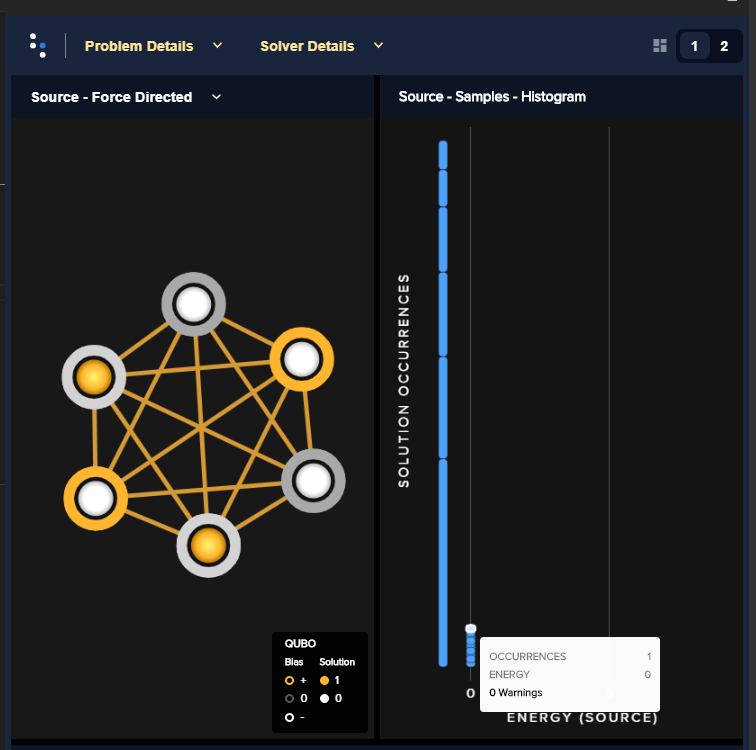# How Do I Read the Histogram in the Problem Inspector?

The histogram gives us a lot of information! Let's look at the simple example below. Here we use the maximum weighted independent set  algorithm to create a QUBO for a complete graph with 6 nodes. We submit the problem to the QPU, receive 500 samples, and print the resulting sample set.There are a couple important things to note in image above. The first is that when we printed out the response we got 23 unique solutions in the sample set. Since the QPU provides probabilistic solutions, we need to take a bunch of reads when we solve a problem. Though it's slightly difficult to see from the image, there are 23 blocks in the histogram, which correspond to the 23 unique solutions. The histogram shows

1. How many distinct solutions there are in a sample set (the number of bins)
2. How many occurrences of each solution there are (height of the bin)
3. What the energy is for each distinct solution (position on the x-axis)

We can display different solutions in the graph by selecting different bins in the histogram. Here's an example.One last thing to note is that if you hover over a bin in the histogram a pop up will display the number of occurrences, energy and warnings for that solution.

Here are some related resources on using the QPU: